Scan

Suppose we have a list of expressions which in the example below is a simple
list of integers.  Now suppose we need to define a function (f) for each
element of this list. The best way to make the assignments is using Scan.  A
first attempt at this is given in the next cell.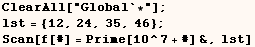Next we see that our attempt to make assingments for (f) didn't work, and the
reason is that assignment (f[#]=Prime[10^7+#]&) evaluated before it was give
integers.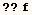Global`f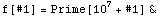The solution is to make the assignment a function with the HoldAll or
HoldFirst attribute as I do in the next cell.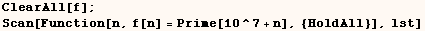Global`f

 f = 179424871 f = 179425019 f = 179425261 f = 179425517

We could have made the above assingments using Map as I do in the next cell.
In this case Map makes a list of prime numbers that would be returned if it
were not for the semi-colon at the end.  Using Scan for this task is more
efficient than using Map because Scan never builds up an expression to
return.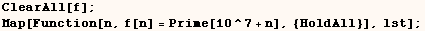Global`f

 f = 179424871 f = 179425019 f = 179425261 f = 179425517

The next example makes assingments for  f[g1[5,3]],  f[g2[8,9]],  and
f[g3[12,13]].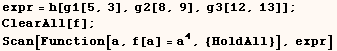Global`f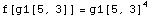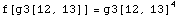However, what we got above might not be the desired result. What if you  wanted to make assignments for f, f, f, etc. In that case we can get  the desired result by giving Scan the level specification {2}.  Scan then  makes assingments for all subexpressions of (expr) at level 2.  Scan can work  with any of the level specifications that I exaplain earlier.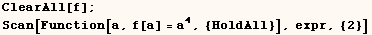Global`f

 f = 81 f = 625 f = 4096 f = 6561 f = 20736 f = 28561

Heads Option

Scan has a Heads option with the default setting (Heads→True).  In the
next example I have Scan work on level {2} of expr with the setting (Heads
→True).  Because of the (Heads→True) setting assignments are made
for f[g1], f[g2], f[g3] where g1, g2, g3 are heads of sub-expressions of
(expr).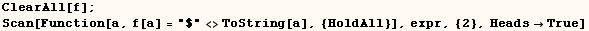Global`f

 f = \$3 f = \$5 f = \$8 f = \$9 f = \$12 f = \$13 f[g1] = \$g1 f[g2] = \$g2 f[g3] = \$g3

Created by Mathematica  (May 16, 2004)

Back to Ted’s Tricks index page